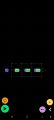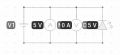# The most basic circuit i couldve ever made.. is shorted

#### catinin

Joined Aug 22, 2023
1so, I was messing around in an app called PROTO (I know nothing about electricity,I'm just messing around) and..shorted voltage source. Only affected me when I used an ammeter. I tried putting it in a parallel circuit, doesn't work. Can someone explain?? AC power type, and thats an LED on the right.

#### djsfantasi

Joined Apr 11, 2010
9,125
An ammeter should be placed in series between the power supply (or virtual ground. Nevermind about that for now) and the circuit being measured. Your simple circuit has it in parallel. In parallel, it will be a dead short.

Place it in the top replacing the wire to the LED.

#### crutschow

Joined Mar 14, 2008
33,325
Obviously you need to do a little further study on electricity basics.

The current through an LED is in the forward direction, so its anode must be positive with respect to the negative.
And it needs a resistor in series to establish the desired current through the LED.

#### LowQCab

Joined Nov 6, 2012
3,559
Current flows only in a "Circuit", around and around.
This means that an Ammeter must be installed "in-series" with the Circuit, not "in-parallel" with the Battery.

Voltage is measured between 2 different points in a Circuit.

An Ammeter can be placed anywhere in a Circuit,
since the Current is the same everywhere in any Circuit.
But, there may be more than one Circuit connected to the same Battery.

An Ammeter may be treated as a piece of Wire with zero Resistance,
( all of the Current flowing in the Circuit will flow through it without being altered ).

But, a Voltmeter is treated as an infinite Resistance, ( no Current will flow through it ).
.
.
.

#### MrChips

Joined Oct 2, 2009
29,801
Welcome to AAC!

You need to begin with a solid knowledge and understanding of Ohm's Law.

Ohm's Law is stated algebraically as:

I = V / R

Where,
I = current (amps)
V = voltage (volts)
R = resistance (ohms)

Two corollaries (i.e. variants) of Ohm's Law are:

V = I x R
R = V / I

Once you have mastered Ohm's Law, you will come to appreciate that if R = 0, I = infinite:

I = V / 0 = ∞

Thus, you need finite resistance in every circuit otherwise you get infinite currents flowing.
An ammeter measures current flow in a circuit. Hence an ammeter has to measure current flowing through itself.
Go figure what that means on where to connect an ammeter. In other words, an ammeter will measure the current in any branch circuit into which it is inserted.

#### dl324

Joined Mar 30, 2015
16,110
I was messing around in an app called PROTO (I know nothing about electricity,I'm just messing around) and..shorted voltage source. Only affected me when I used an ammeter.
Here's an easier-to-read schematic (you should space the components so they don't have overlapping text):An ideal current meter has 0 internal resistance, so it's shorting the power supply.

Aside from the fact that the LED is wired backwards, you need a current limiting resistor.

Since you don't know anything about electricity, I'd suggest that you learn some basics before trying to design any circuits.